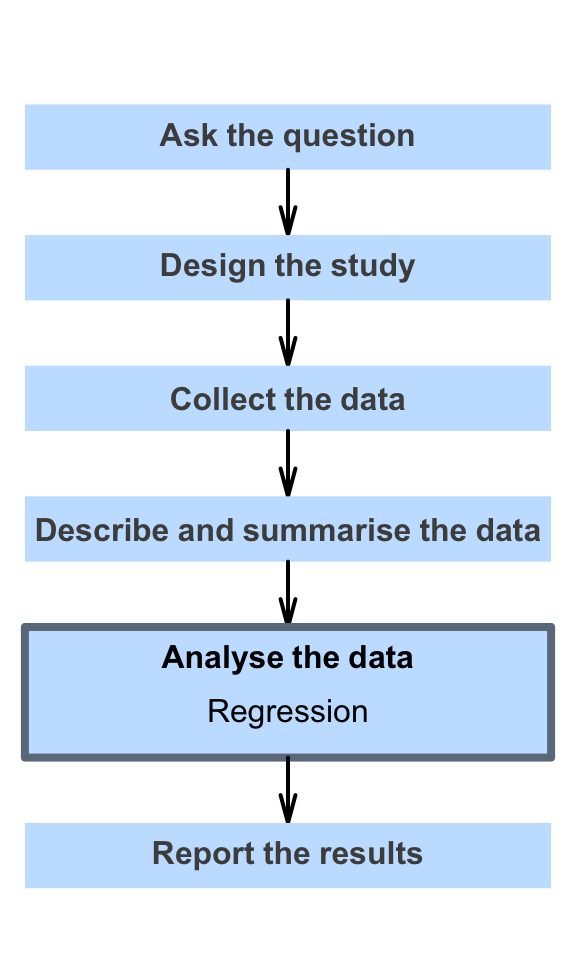# 35 Regression

So far, you have learnt to ask a RQ, identify different ways of obtaining data, design the study, collect the data describe the data, summarise data graphically and numerically, understand the tools of inference, to form confidence intervals, and to perform hypothesis tests.

In this chapter, you will learn about regression. You will learn to:

• produce and interpret linear regression equations.
• conduct hypothesis tests for the slope in a regression line.
• produce confidence intervals for the slope in a regression line.